# IB DP Physics: SL复习笔记4.2.3 Electromagnetic Waves

### Electromagnetic Waves

• An electromagnetic wave is generated by the combined oscillation of an electric and a magnetic field
• These fields oscillate perpendicular to each other and to the direction of motion of the wave (i.e. the direction in which energy is transferred)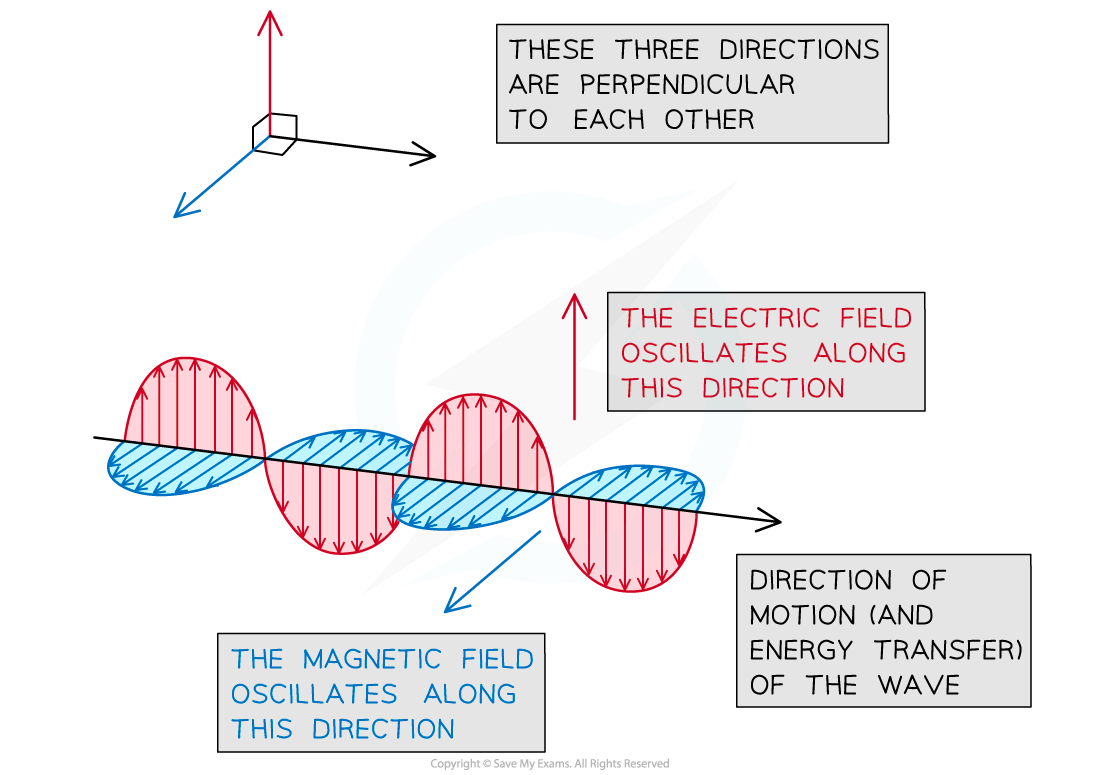An electromagnetic wave is generated by the combined oscillation of an electric and a magnetic field

• Electromagnetic waves are transverse waves and, as such, they can travel through vacuum
• Regardless of their frequency, all electromagnetic waves travel at the speed of light c = 3 × 108 m s–1 in vacuum
• Electromagnetic waves form a continuous spectrum based on their frequencyThe electromagnetic spectrum

• Humans can only sense electromagnetic waves with wavelengths in the range 700 nm - 400 nm, which are the limits of the so-called visible spectrum
• Electromagnetic waves with longer and shorter wavelengths are invisible to the human eye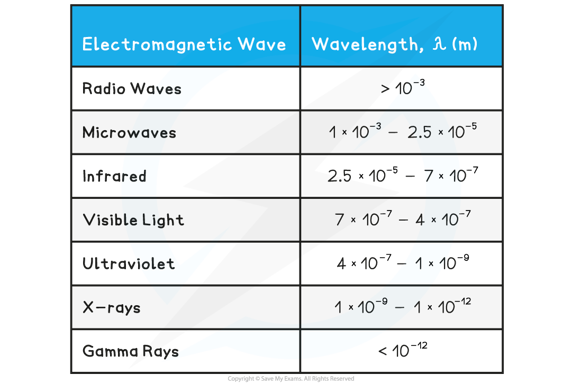• Knowing the wavelengths of electromagnetic waves, their frequencies can be calculated using the wave equation, and remembering that their speed in vacuum is always equal to the speed of light

#### Worked Example

The wavelength of blue light falls within the range 450 nm - 490 nm. Determine the range of frequencies of blue light.

Step 1: Write down the known quantities

• Note that you must convert the values of the wavelength from nanometres (nm) into metres (m)
• λlower = 450 nm = 4.5 × 10–7 m
• λhigher = 490 nm = 4.9 × 10–7 m

Step 2: Remember that all electromagnetic waves travel at the speed of light in vacuum

• From the data booklet, c = 3 × 108 m s–1

Step 3: Write down the wave equation

c = fλ

Step 4: Rearrange the above equation to calculate the frequency f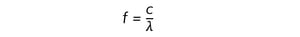Step 5: Substitute the lower and higher values of the wavelength to calculate the limiting values of the frequency of blue light

• The lower frequency flower corresponds to the higher value of the wavelength λhigher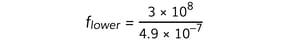= 6.1 × 1014 Hz

• The higher frequency fhigher corresponds to the lower value of the wavelength λlower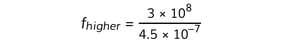= 6.7 × 1014 Hz

Step 6: Write down the range of frequencies of blue light

f = 6.1 × 1014 Hz - 6.7 × 1014 Hz

#### Exam Tip

You must memorise all electromagnetic waves in the correct order, as well as the range of wavelengths for each type of wave. You must also remember that all electromagnetic waves travel at the speed of light in vacuum.